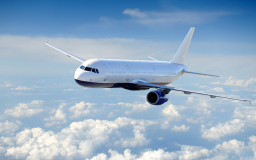# Fu Da

Fu Da spent a whole day traveling. 5/8 of the journey was spent by airplane, and 1/4 of the remainder was by train. He spent 6 3/4 h on the bus journey.

a) How long did Fu Da spend on the train journey?
b) How much longer did Fu Da spend on the airplane than on the bus?

t =  2 14 = 9/4 h
y =  8 14 = 33/4 h

### Step-by-step explanation:Did you find an error or inaccuracy? Feel free to write us. Thank you!

Tips for related online calculators
Need help calculating sum, simplifying, or multiplying fractions? Try our fraction calculator.
Need help with mixed numbers? Try our mixed-number calculator.
Do you have a linear equation or system of equations and looking for its solution? Or do you have a quadratic equation?
Do you want to convert time units like minutes to seconds?